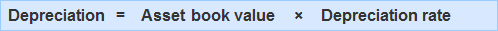## Reducing Balance Method: Definition

Under the reducing balance method, the amount of depreciation is calculated by applying a fixed percentage on the book value of the asset each year.

In this way, the amount of depreciation each year is less than the amount provided for in the previous year. This is because the book value used to compute the depreciation expense is continually reduced from year to year.

## Reducing Balance Method: Formula

Use the following formula to calculate depreciation under the reducing balance method:Depreciation = Asset book value x Depreciation rate

Where:

Depreciation is the dollar amount lost in value

Asset book value is the value of the asset for accounting purposes

Depreciation rate is the percentage decline in the asset’s value

## Example: Calculating Depreciation Under Reducing Balance Method

On 1 January 2016, XYZ Limited purchased a truck for \$75,000. Depreciation is estimated at 20% per year on the book value.

Required: Calculate the truck’s depreciation for 2016, 2017, and 2018.

### Solution

#### 2016

The book value at the beginning of 2016 is \$75,000. Depreciation for 2016 is \$75,000 × 0.2 = \$15,000.

#### 2017

The book value at the beginning of 2017 is \$75,000 – \$15,000 = \$60,000. Depreciation for 2017 is \$60,000 × 0.2 = \$12,000.

#### 2018

The book value at the beginning of 2018 is \$60,000 – \$12,000 = \$48,000. Depreciation for 2018 is \$48,000 × 0.2 = \$9,600.

#### Notes

Notice that the depreciation provided in 2018 (\$9,600) is less than the amount of depreciation provided in 2017 (\$12,000). In turn, this is less than the amount provided in 2016 (\$15,000).

The reason for this is that the rate of depreciation (20% in this case) is being applied to the book value, which continually reduces each year.

In 2016, the book value was \$75,000, while in 2017, it fell to \$60,000. A year later, it reduced to \$48,000. Remember that the rate of depreciation remains constant but it is applied to a lesser amount (i.e., book value) each year.

Hence, the amount of depreciation each year is lower.

The reducing balance method is also known as the reducing installment method. It is especially useful for fixed assets whose value deteriorates faster in the earlier years of usage (e.g., cars, office equipment, and small machinery).

### What is the definition of the reducing balance method?

Under the reducing balance method, the amount of depreciation is calculated by applying a fixed percentage on the book value of the asset each year.

### What are the factors that impact the book value of an asset?

The book value is directly related to factors such as useful life, wear and tear, availability of spare parts for repairs, ability to resell at market price should it be necessary to dispose of the asset etc.

### How can you calculate the book value?

By using this formula: asset cost - accumulated depreciation = book value

### How does the reducing balance method differ from the straight-line method?

The key difference between these two methods is their computation of depreciation expense. Under the straight line method, depreciation is provided evenly over the lifetime of an asset at a constant rate. This means that an equal amount will be deducted each year regardless of when it was purchased.

### What is the reducing balance method formula?

Depreciation = asset book value x depreciation rate

Disclaimer: The above references an opinion and is for information purposes only. It is not intended to be investment advice. Seek a duly licensed professional for investment advice.

True is a Certified Educator in Personal Finance (CEPF®), a member of the Society for Advancing Business Editing and Writing, contributes to his financial education site, Finance Strategists, and has spoken to various financial communities such as the CFA Institute, as well as university students like his Alma mater, Biola University, where he received a bachelor of science in business and data analytics.

To learn more about True, visit his personal website, view his author profile on Amazon, his interview on CBS, or check out his speaker profile on the CFA Institute website.